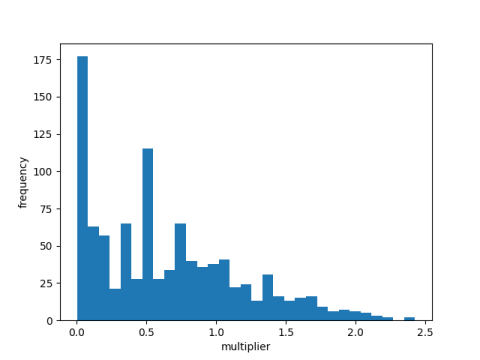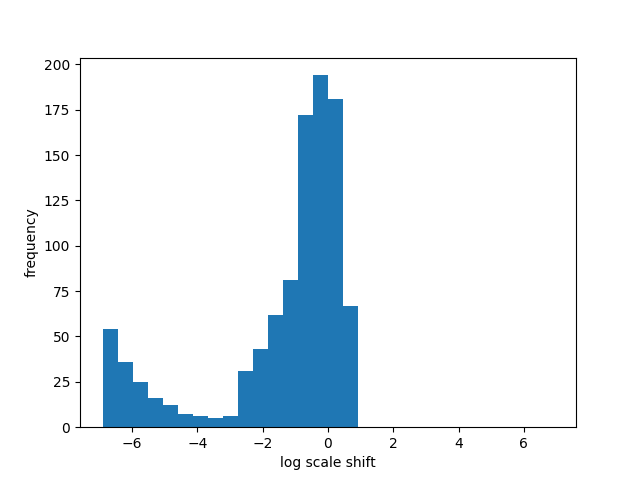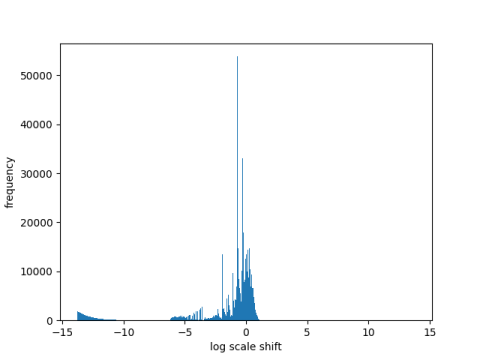# Aliquot ratio distribution

The previous post looked at repeatedly applying the function s(n) which is the sum of the divisors of n less than n. It is an open question whether the sequence

s( s( s( … s(n) … ) ) )

always converges or enters a loop. In fact, it’s an open question of whether the sequence starting with n = 276 converges.

A heuristic argument for why the sequence ought to converge, at least much of the time, is that s(n) is usually less than n. This can be made precise as explained here: in the limit as N goes to infinity, the proportion of numbers less than N for which s(n) < n is at least 0.752.

Even though applying s usually leads to a smaller result, conceivably it could lead to a much larger result when it increases, as with the hailstone problem.

I made some histograms of the ratio s(n) / n to get a feel for how much s increases or decreases its argument. (I imagine this has all been done before, but I haven’t seen it.)

Here’s my first attempt, for n up to 1000.So apparently the ratio is often less than 1.

I thought it would be clearer to work on a log scale and center the graph at 0 so I next made the following graph.This shows that the aliquot function s never increases its argument by much, and it often reduces its argument quite a bit, at least for numbers less than 1000.

Next I made a histogram with numbers less than 1,000,000 to see whether the pattern continued. Here’s what I got.The most obvious feature is the huge spike. If you can ignore that, mentally cropping the image to just the bottom part, it looks basically like the previous graph.

What’s up with that spike? It’s in the bin containing log(0.5). In other words, the ratio s(n)/n is often near 0.5.

So I thought maybe there’s some number theoretic reason the ratio is often exactly 1/2. That’s not the case. It’s only exactly 1/2 once, when n = 2, but it’s often near 1/2.

In any case, applying s very often returns a smaller number than you started with, so it’s plausible that the iterated aliquot sequence would often converge. But empirically it looks like some sequences, such as the one starting at n = 276, diverge. They somehow weave through all the numbers for which the sequence would come tumbling down.